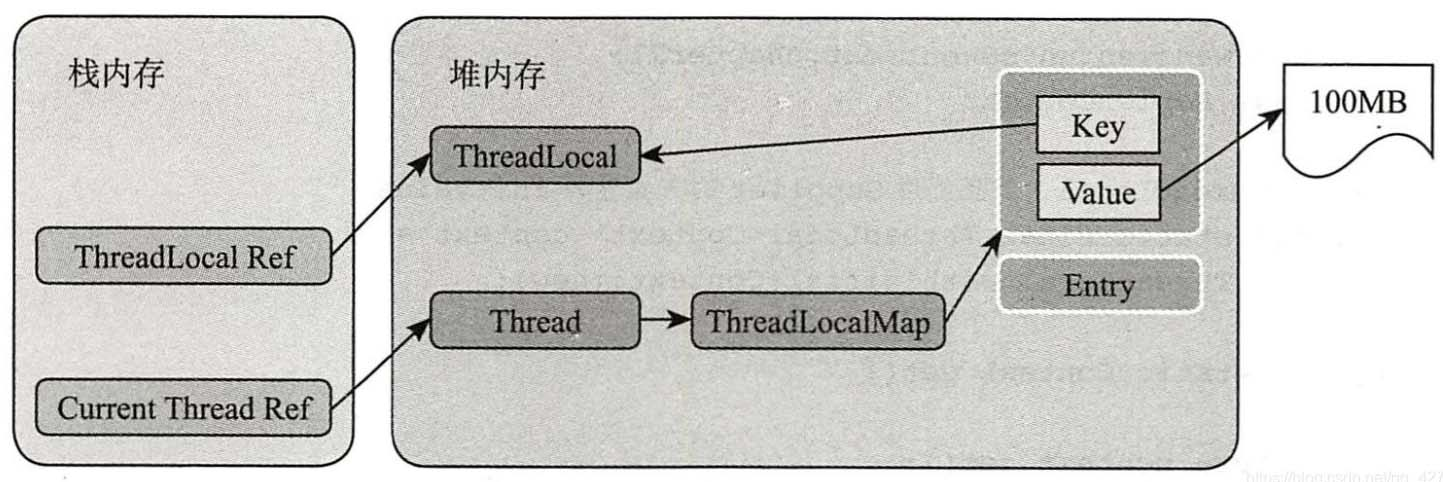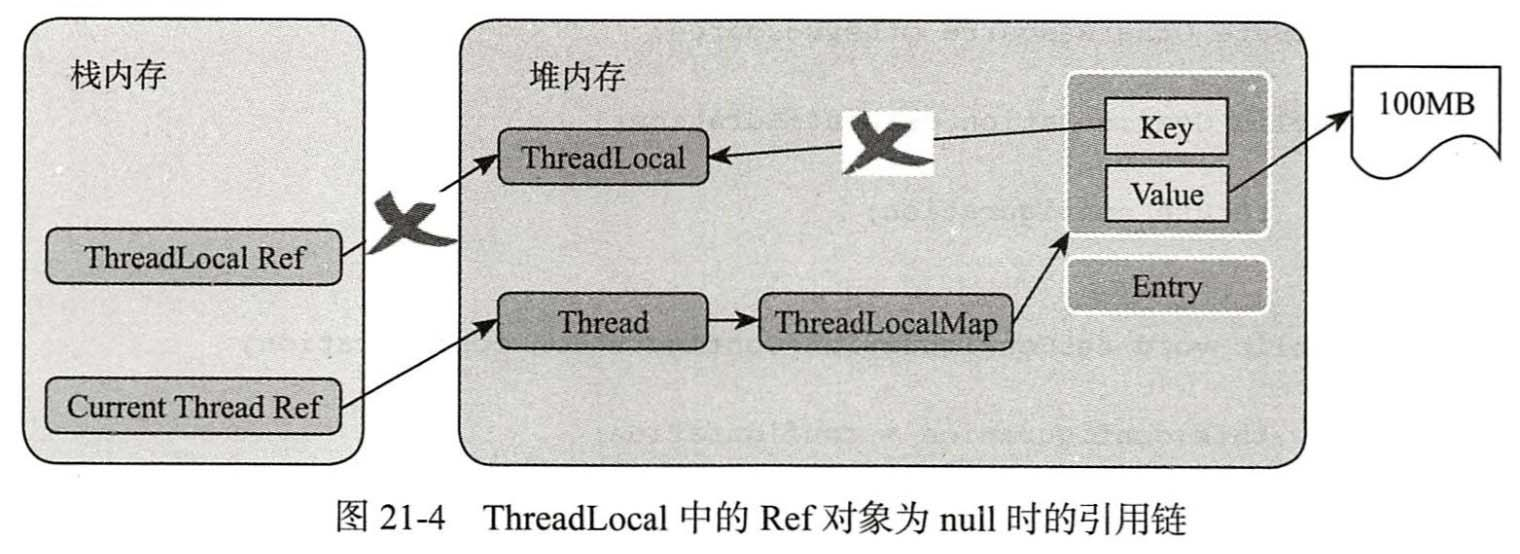##### 目录
• 3.常用方法源码解析
• 3.1 initialValue方法
• 3.2 set(T value)方法
• 3.3 get方法
• 3.4 小结

• 线程间数据隔离。
• 进行事务操作，用于储存线程事务信息。

注意：

## 3.常用方法源码解析

### 3.1 initialValue方法

``` protected T initialValue() {
return null;
}```

如果使用的是Java8提供的Supplier函数接口更加简化：

```// withInitial()实际是创建了一个ThreadLocal的子类SuppliedThreadLocal，重写initialValue()

### 3.2 set(T value)方法

主要存储指定数据。

```public void set(T value) {
if (map != null)
// 核心方法。set 遍历整个Entry的过程，后面有详解
map.set(this, value);
else {
// 当前线程的hashCode&(entry默认长度-1)),并设置阈值(默认为0)为Entry默认长度的2/3。
createMap(t, value);
}
}
// set 遍历整个Entry的过程
private void set(ThreadLocal<?> key, Object value) {
// 获取所有的Entry
Entry[] tab = table;
int len = tab.length;
int i = key.threadLocalHashCode & (len-1);
// 循环查找
for (Entry e = tab[i];e != null;e = tab[i = nextIndex(i, len)]) {
// 找到相同的就直接覆盖，直接返回。
if (k == key) {
e.value = value;
return;
}
// 这个过程主要是防止内存泄漏。
if (k == null) {
replaceStaleEntry(key, value, i);
return;
}
}
tab[i] = new Entry(key, value);
int sz = ++size;
if (!cleanSomeSlots(i, sz) && sz >= threshold)
rehash();
}
```

### 3.3 get方法

```public T get() {
if (map != null) {
// 而此Entry是放在一个Entry数组里面的。
if (e != null) {
// 直接返回当前数据
T result = (T)e.value;
return result;
}
}
return setInitialValue();
}
private T setInitialValue() {
T value = initialValue();
if (map != null)
// 如果map存在，直接调用set()方法进行赋值。
map.set(this, value);
else
createMap(t, value);
return value;
}
```

### 3.4 小结

• `initialValue()`：初始化ThreadLocal中的value属性值。
• `set()`：获取当前线程，根据当前线程从ThreadLocals中获取ThreadLocalMap数据结构，
• `get()`

线程复用会产生脏数据。

```// java.lang.Thread#threadLocals
* by the ThreadLocal class. */
```

在get数据的时候，增加检查，清除已经被回收器回收的Entry（WeakReference可以自动回收）

```private Entry getEntryAfterMiss(ThreadLocal<?> key, int i, Entry e) {
...
if (k == null)
// 清除 key 是 null 的Entry
expungeStaleEntry(i);
...
return null;
}
private boolean cleanSomeSlots(int i, int n) {
boolean removed = false;
Entry[] tab = table;
int len = tab.length;
do {
i = nextIndex(i, len);
Entry e = tab[i];
if (e != null && e.get() == null) {
n = len;
removed = true;
// 清除key==null 的Entry
i = expungeStaleEntry(i);
}
} while ( (n >>>= 1) != 0);
return removed;
}
```

set数据时增加检查，删除已经被垃圾回收器清理的Entry，并将其移除

```private boolean cleanSomeSlots(int i, int n) {
boolean removed = false;
Entry[] tab = table;
int len = tab.length;
do {
i = nextIndex(i, len);
Entry e = tab[i];
if (e != null && e.get() == null) {
n = len;
removed = true;
// 清除key==null 的Entry
i = expungeStaleEntry(i);
}
} while ( (n >>>= 1) != 0);
return removed;
}```5

```public void remove() {
if (m != null)
// map不为null，执行remove操作
m.remove(this);
}
// 获取存放key-value的数组。
Entry[] tab = table;
int len = tab.length;
int i = key.threadLocalHashCode & (len-1);
for (Entry e = tab[i];e != null;e = tab[i = nextIndex(i, len)]) {
if (e.get() == key) {
e.clear();
// 实行Enty和Entry.value置null。源码中 tab[staleSlot].value = null; tab[staleSlot] = null;
expungeStaleEntry(i);
return;
}
}
}
```

以上为个人经验，希望能给大家一个参考，也希望大家多多支持自由互联。

上一篇：初识Java一些常见的数据类型
下一篇：没有了
网友评论
相关栏目
﻿